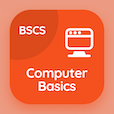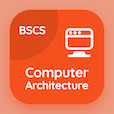Online CS Degree Courses

Digital Logic Design Quizzes

Digital Logic Design Quiz - Complete

Practice Two Level Implementations quiz questions and answers, two level implementations MCQ questions PDF, test 108 to study Digital Logic Design online course. Simplification of Boolean Functions MCQ questions, two level implementations Multiple Choice Questions (MCQ Quiz) for online college degrees. Two Level Implementations Book PDF: gray code, asm chart, flip-flops, combinational circuits, two level implementations test prep for free online classes.

"The K-map based Boolean reduction is based on the following Unifying Theorem: A + A' = 1" Quiz PDF: two level implementations App APK with non impact, impact, force, and complementary choices for computer majors. Solve simplification of boolean functions questions and answers to improve problem solving skills for best online schools for computer science.

## Trivia Questions on Two Level Implementations MCQs

MCQ: The K-map based Boolean reduction is based on the following Unifying Theorem: A + A' = 1

impact
non impact
force
complementary

MCQ: 3x8 decoder will have

4outputs
5outputs
6outputs
8outputs

MCQ: The basic latch consists of

Two inverters
Two comparators
Two amplifiers

MCQ: State box has a shape of

square
rectangle
rhombus
pentagon

MCQ: Gray code representation of 14 is

1010
1100
1001
1110

### More Quizzes from Digital Logic Design Book